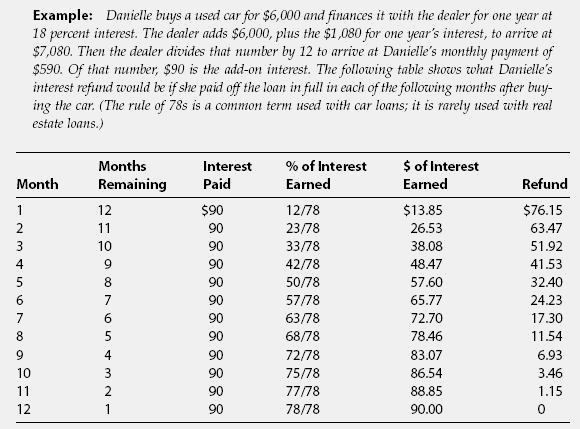# rule of 78s

Also found in: Legal, Wikipedia.

## Rule of 78

A practice in which lenders amortize repayment of short-term loans in a way that the borrower pays most of the interest earlier. For example, in a 12-month loan, the borrower will pay nearly all of the interest over the first, say, six or seven months before his/her payments cover any principal at all. The Rule of 78 guarantees that the lender will still make a profit if the borrower repays the loan early. However, it does not do anything to protect the borrower and is illegal to use for loans with a term longer than 61 months.

## rule of 78sA method for computing the refund due when a borrower wants to pay off an add-on interest loan at an earlier time than the maturity date. The reason there would be any refund at all is because all the interest is calculated up front, for the entire loan, and then paid in equal monthly installments. If the loan is repaid early, some portion of the interest has not been earned, but it has already been paid. Use of the rule of 78s results in the lender “earning” more of the interest than one might otherwise think. To calculate, you need to use a fraction that changes every month. For the first month,the numerator (top number) is the number of months remaining in the loan. The denominator (bottom number) is the sum of the digits for the number of months of the original loan.If the loan is for 1 year, then you add 1+ 2 + 3 + 4 + … + 11 + 12 = 78. For the first month, with 12 months remaining in the loan, the fraction is 12/78. According to the method, 12/78 of the interest has been earned in the first month, or 15.38 percent.You might think that only 1/12 of the interest—8.33 percent—has been earned,since only one month has passed,but that's the way the rule of 78s works— to the lender's advantage. The next month, you change the numerator by adding the number of remaining months (11) to last month's numerator of 12.The numerator is then 11 + 12 = 23, so 23/78 of the interest has been earned, or 29.48 percent. For month 3, the numerator is 10 + 23, and so on.

Mentioned in ?
References in periodicals archive ?
In comparison, under the rule of 78s the borrower is considered to have paid \$15.
Rule Of 78s Month Cumulative Interest Payments Simple Rule Of 78s Interest 1 \$ 15.
The Rule of 78s method allocates interest over the term of a loan based, in part, on the sum of the periods' digits for the loan term.
Based on this opinion, Price continued to apply the rule of 78s through 1988.
The Board testified on a similar bill in the Senate in 1979 and continues to believe that the sum of the digits, or Rule of 78s, method for rebating unearned finance charges may be less fair to consumers who prepay longer-term loans in early years than other methods, such as rebates calculated according to the actuarial method.
Under the Rule of 78s method, the finance charge is earned faster than under the actuarial method.
More than half of the states have either abolished or restricted the use of the Rule of 78s rebate method.

Site: Follow: Share:
Open / Close